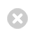Close

MPHY0021: Research Software Engineering With Python

Home## Functions¶

### Definition¶

We use def to define a function, and return to pass back a value:

In :
def double(x):
return x * 2

print(double(5), double(), double('five'))

10 [5, 5] fivefive


### Default Parameters¶

We can specify default values for parameters:

In :
def jeeves(name = "Sir"):
return f"Very good, {name}"

In :
jeeves()

Out:
'Very good, Sir'
In :
jeeves('John')

Out:
'Very good, John'

If you have some parameters with defaults, and some without, those with defaults must go later.

If you have multiple default arguments, you can specify neither, one or both:

In :
def jeeves(greeting="Very good", name="Sir"):
return f"{greeting}, {name}"

In :
jeeves()

Out:
'Very good, Sir'
In :
jeeves("Hello")

Out:
'Hello, Sir'
In :
jeeves(name = "John")

Out:
'Very good, John'
In :
jeeves(greeting="Suits you")

Out:
'Suits you, Sir'
In :
jeeves("Hello", "Producer")

Out:
'Hello, Producer'

### Side effects¶

Functions can do things to change their mutable arguments, so return is optional.

This is pretty awful style, in general, functions should normally be side-effect free.

Here is a contrived example of a function that makes plausible use of a side-effect

In :
def double_inplace(vec):
vec[:] = [element * 2 for element in vec]

z = list(range(4))
double_inplace(z)
print(z)

[0, 2, 4, 6]

In :
letters = ['a', 'b', 'c', 'd', 'e', 'f', 'g']
letters[:] = []


In this example, we're using [:] to access into the same list, and write it's data.

vec = [element*2 for element in vec]



would just move a local label, not change the input.

But I'd usually just write this as a function which returned the output:

In :
def double(vec):
return [element * 2 for element in vec]


Let's remind ourselves of the behaviour for modifying lists in-place using [:] with a simple array:

In :
x = 5
x = 7
x = ['a', 'b', 'c']
y = x

In :
x

Out:
['a', 'b', 'c']
In :
x[:] = ["Hooray!", "Yippee"]

In :
y

Out:
['Hooray!', 'Yippee']

### Early Return¶

Return without arguments can be used to exit early from a function

Here's a slightly more plausibly useful function-with-side-effects to extend a list with a specified padding datum.

In :
def extend(to, vec, pad):
if len(vec) >= to:
return # Exit early, list is already long enough.

vec[:] = vec + [pad] * (to - len(vec))

In :
x = list(range(3))
extend(6, x, 'a')
print(x)

[0, 1, 2, 'a', 'a', 'a']

In :
z = range(9)
extend(6, z, 'a')
print(z)

range(0, 9)


### Unpacking arguments¶

If a vector is supplied to a function with a '*', its elements are used to fill each of a function's arguments.

In :
def arrow(before, after):
return f"{before} -> {after}"

arrow(1, 3)

Out:
'1 -> 3'
In :
x = [1, -1]
arrow(*x)

Out:
'1 -> -1'

This can be quite powerful:

In :
charges = {"neutron": 0, "proton": 1, "electron": -1}
for particle in charges.items():
print(arrow(*particle))

neutron -> 0
proton -> 1
electron -> -1


### Sequence Arguments¶

Similiarly, if a * is used in the definition of a function, multiple arguments are absorbed into a list inside the function:

In :
def doubler(*sequence):
return [x * 2 for x in sequence]

In :
doubler(1, 2, 3)

Out:
[2, 4, 6]
In :
doubler(5, 2, "Wow!")

Out:
[10, 4, 'Wow!Wow!']

### Keyword Arguments¶

If two asterisks are used, named arguments are supplied inside the function as a dictionary:

In :
def arrowify(**args):
for key, value in args.items():
print(f"{key} -> {value}")

arrowify(neutron="n", proton="p", electron="e")

neutron -> n
proton -> p
electron -> e


These different approaches can be mixed:

In :
def somefunc(a, b, *args, **kwargs):
print("A:", a)
print("B:", b)
print("args:", args)
print("keyword args", kwargs)

In :
somefunc(1, 2, 3, 4, 5, fish="Haddock")

A: 1
B: 2
args: (3, 4, 5)